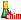﻿ Metal corrosion# Metal corrosion

## Local batteries

The seemingly simple reaction of zinc corrosion by acid $Zn+2H^+$ $\rightarrow$ $Zn^{2+}+H_2$ poses many understanding problems : 1) Is it possible that positive Zn and H ions continuously diffuse in opposite directions, the $Zn^{2+}$ ions from the solid zinc to the solution, the $H^+$ ions from the solution to the solid zinc ? Physics teaches us that positive charges always move in one direction in an electric field! 2) How is it that the reaction is much faster with impure zinc (containing traces of other metals) than with highly pure zinc? 3) How can it be explained that the addition of some copper sulfate significantly increases the speed of reaction (catalyst)? Look at the surface of a piece of zinc contaminated by a copper grain (impurity present from the start or formed by the reaction of $Cu^{2+}$ on $Zn$) and immersed in an acid solution:This system is equivalent to a battery:Indeed, the conductor (short-circuit!) is provided by the zinc itself and the ion $Zn^{2+}$ and molecules $H_2$ will form immediately after transfer of the first electrons, whereas $H^+$ ions, repelled by the $Zn^{2+}$ ion cannot directly attack the zinc (role of the semipermeable wall in the battery)! The local battery works until exhaustion of zinc or the $H^+$ ions.

## The rusting

Experience shows that water and oxygen are necessary for the formation of rust. Impurities in air ( $CO_2$, $SO_2$, dust) as well as salts in water promote the process. A local cell explains what happens: In regions poor of oxygen, iron goes into solution in the form of iron(II) ion. (Negative electrode of the battery). It leaves 2 electrons that can circulate in the body of the metal ("outer" circuit of the battery). On the surface of the iron, at the places where the solution is oxygen-rich (positive electrode of the battery), the latter picks up electrons to form hydroxide ions with the help of water. Iron(II) ions and hydroxide ions diffuse towards each other in the solution: $Fe^{2 +}(OH^-) _2$ is precipitated. Then, the excess of oxygen produces rust: $4Fe(OH)_2 + O_2$ $\rightarrow$ $2Fe_2O_3.H_2O + 2H_2O$ Rust (iron(II)oxide hydroxide) is porous and makes that the process may continue until the disappearance of the iron.